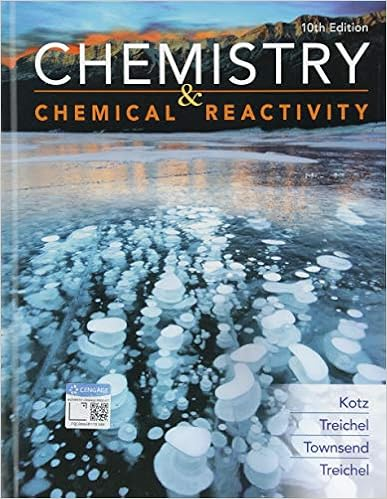# Determine the electron geometry eg and molecular

• Test Prep
• 8
• 100% (3) 3 out of 3 people found this document helpful

This preview shows page 2 - 4 out of 8 pages.

Sample+questions+_chapter+9-10

7 )   Determine the electron geometry ( eg ) and molecular geometry ( mg ) of CO 2 .
A ) eg=tetrahedral , mg=tetrahedral
B ) eg = linear , mg=trigonal planar
C ) eg=trigonal planar , mg=bent
D ) eg =linear , mg=linear
E ) eg=trigonal planar , mg=trigonal planar
10 )   Determine the electron geometry ( eg ) and molecular geometry ( mg ) of NCl 3 .
11 )   Determine the electron geometry ( eg ) and molecular geometry ( mg ) of ICl 3 .
12 )   Determine the electron geometry ( eg ) and molecular geometry ( mg ) of ICl 2 ⁻ .
13 )   Determine the electron geometry ( eg ) and molecular geometry ( mg ) of XeF 2 .
A ) eg=trigonal bipyramidal , mg=bent
B ) eg =linear , mg=linear
C ) eg = tetrahedral , mg=linear
D ) eg=trigonal bipyramidal , mg=linear
E ) eg = tetrahedral , mg = bent
14 )   Determine the electron geometry ( eg ) and molecular geometry ( mg ) of XeF 4 .
15 )   Determine the electron geometry ( eg ) and molecular geometry ( mg ) of the underlined atom H 2 CO .
16 )   Consider the molecule below . Determine the molecular geometry at each of the 2 labeled carbons .
17 )   Consider the molecule below . Determine the molecular geometry at each of the 3 labeled atoms .
A ) 1 = trigonal planar , 2 = tetrahedral , 3= trigonal pyramidal
B ) 1 = tetrahedral , 2=tetrahedral , 3=tetrahedral
C ) 1 = trigonal planar , 2= tetrahedral , 3=tetrahedral
D ) 1= tetrahedral , 2= tetrahedral , 3= trigonal planar
##### We have textbook solutions for you!
The document you are viewing contains questions related to this textbook.The document you are viewing contains questions related to this textbook.
Chapter 9 / Exercise 24
Chemistry & Chemical Reactivity
Kotz/TreichelExpert Verified
7) Determine the electron geometry (eg) and molecular geometry (mg) of CO2. A) eg=tetrahedral, mg=tetrahedral B) eg=linear, mg=trigonal planar C) eg=trigonal planar, mg=bent D) eg=linear, mg=linear E) eg=trigonal planar, mg=trigonal planar Answer:
D 10) Determine the electron geometry (eg) and molecular geometry (mg) of NCl3.
E 11) Determine the electron geometry (eg) and molecular geometry (mg) of ICl3.
B 12) Determine the electron geometry (eg) and molecular geometry (mg) of ICl2.
C 13) Determine the electron geometry (eg) and molecular geometry (mg) of XeF2. A) eg=trigonal bipyramidal, mg=bent B) eg=linear, mg=linear C) eg=tetrahedral, mg=linear D) eg=trigonal bipyramidal, mg=linear E) eg=tetrahedral, mg=bent Answer:
D 14) Determine the electron geometry (eg) and molecular geometry (mg) of XeF4.
##### We have textbook solutions for you!
The document you are viewing contains questions related to this textbook.The document you are viewing contains questions related to this textbook.
Chapter 9 / Exercise 24
Chemistry & Chemical Reactivity
Kotz/TreichelExpert Verified
E
•••